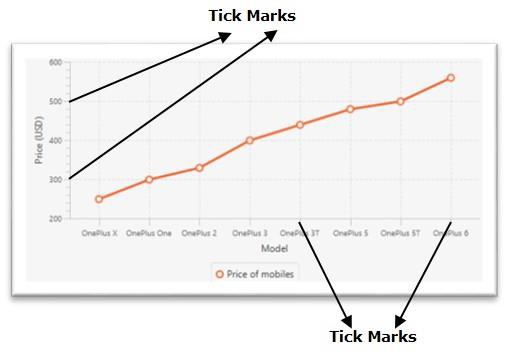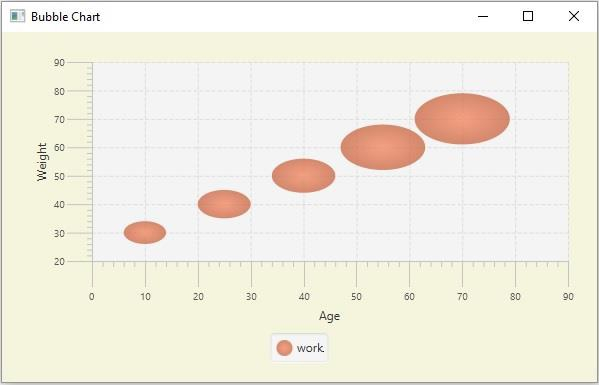# How to modify the length of the tick marks in JavaFX XY charts?

JavaFXObject Oriented ProgrammingProgramming

The javafx.scene.XYChart class is the base class of all the charts that are plotted in an x-y pane. By instantiating the subclasses of this class you can create various XY charts namely − line chart, area chart, bar chart, pie chart, bubble chart, scatter chart, etc.

In the XY chart, the given data points are plotted on an XY plane. Along the x and y axes, you will have the tick marks and tick labels. The tick marks represent various values with uniform intervals.## Changing the length of the tick marks

The javafx.scene.chart.Axis class (abstract) is the base class of all the axes in XY charts. To create X and Y axes you need to instantiate subclasses of these classes

The NumberAxis class is used to create an axis for numerical values and the CategoryAxis class is used to create axis for string categories.

This class has a property named tick length (double), it specifies the length of all the tick lines in the current axis. You can set the value of this property using the setTickLength() method.

To change the length of the tick marks of an XY chart invoke this method bypassing the required length as a parameter.

## Example

import javafx.application.Application;
import javafx.geometry.Insets;
import javafx.scene.Scene;
import javafx.scene.chart.BubbleChart;
import javafx.stage.Stage;
import javafx.scene.chart.NumberAxis;
import javafx.scene.chart.XYChart;
import javafx.scene.layout.StackPane;
public class TickMarkLength extends Application {
public void start(Stage stage) {
//Creating X and Y axes
NumberAxis xAxis = new NumberAxis(0, 90, 10);
NumberAxis yAxis = new NumberAxis(20, 90, 10);
//Creating labels to the axes
xAxis.setLabel("Age");
yAxis.setLabel("Weight");
//Creating the Bubble chart
BubbleChart bubbleChart = new BubbleChart(xAxis, yAxis);
//Preparing data for bubble chart
XYChart.Series series = new XYChart.Series();
//Setting the data to bar chart
//Setting name to the bubble chart
series.setName("work");
//Changing the length of the tickmark
xAxis.setTickLength(25);
yAxis.setTickLength(25);
//Creating a stack pane to hold the chart
StackPane pane = new StackPane(bubbleChart);
pane.setStyle("-fx-background-color: BEIGE");
//Setting the Scene
Scene scene = new Scene(pane, 595, 350);
stage.setTitle("JavaFX Example");
stage.setScene(scene);
stage.show();
}
public static void main(String args[]){
launch(args);
}
}

## Output